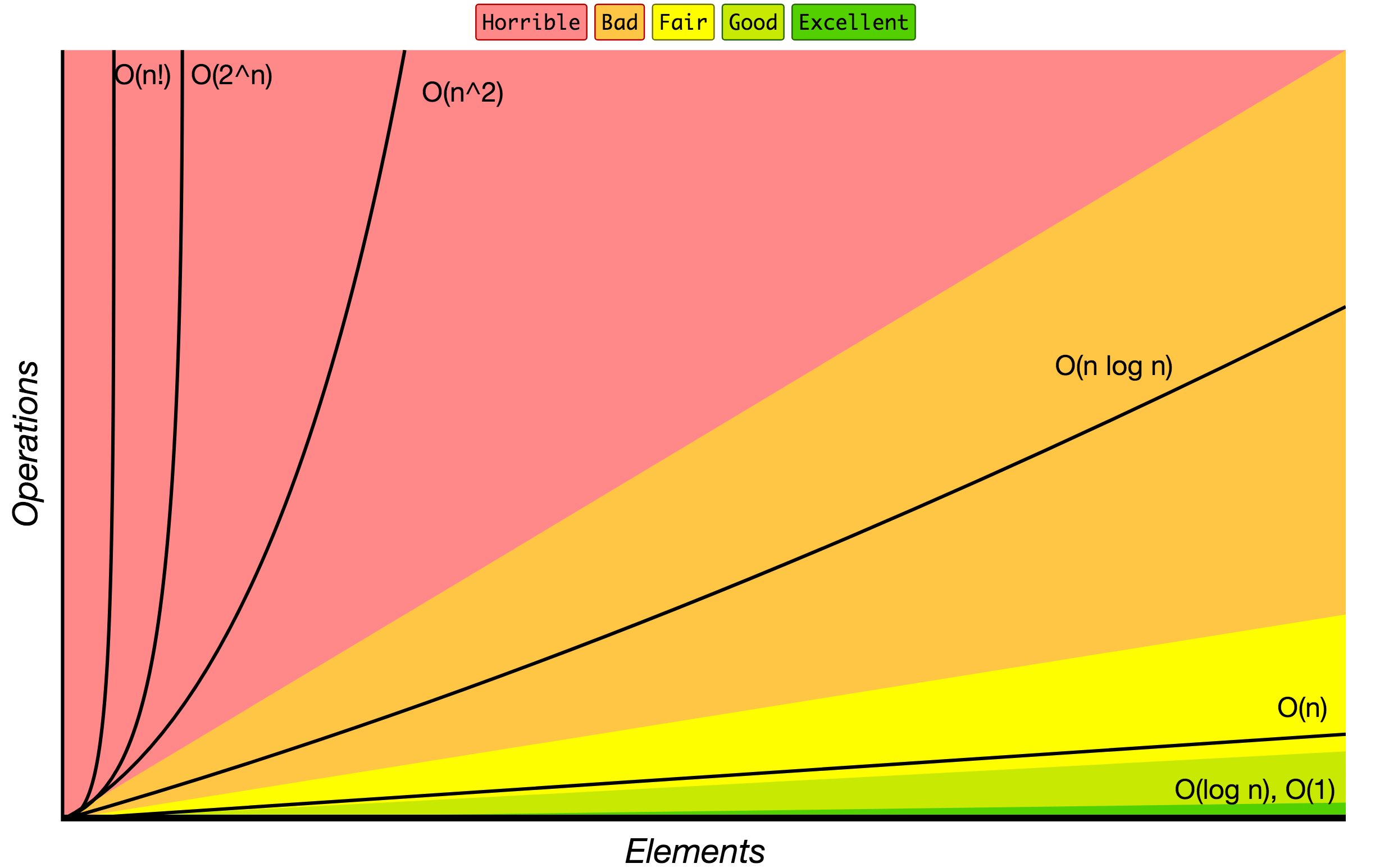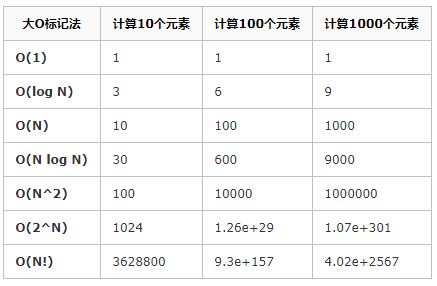• 理解程序执行的时间复杂度和空间复杂度对于优化程序非常重要 本篇文章重点分析如何计算一个程序时间复杂度和空间复杂度 一、时间复杂度 对涉及的对数时间复杂度写法的说明： 平时我们计算时间复杂度常说的logn...
理解程序执行的时间复杂度和空间复杂度对于优化程序非常重要
本篇文章重点分析如何计算一个程序的时间复杂度和空间复杂度
一、时间复杂度
对涉及的对数时间复杂度写法的说明：
平时我们计算时间复杂度常说的logn以及nlog都没有涉及底数，这是因为无论是以e为底，还是以某个常数为底，底数始终是一个常数，在计算时间复杂度时常数可以忽略，因此，习惯性的写为logn、nlogn等等。
概念
执行算法所需要消耗的时间长短，称为算法的时间复杂度。
时间复杂度的表示方式(大O)
T(n)=O(f(n))
n是影响复杂度变化的因子，f(n)是复杂度具体的算法。
常见的时间复杂度量级(从小到大)
常数阶:O(1)；
对数阶:O(logn)
线性阶:O(n)；
线性对数阶:O(nlogn)
平方阶:O(n2)
立方阶:O(n3)
k次方阶:O(nk)
指数阶:O(2n)
阶乘阶:O(n!)
下面为了方便理解这些阶数的大小，给出博客(https://www.cnblogs.com/chenjinxinlove/p/10038919.html)
中绘制的时间复杂度曲线以及性能比较。举例分析
常数阶
int x=1;
int y=7;
y=x;

时间复杂度表示的是代码执行时间的一个增长变化趋势，在上面的代码中无论出现多少个赋值语句，其时间复杂度仍为O(1)。这是由于赋值操作本身没有增长趋势。
对数阶
int i=1;
while(i<n){
i*=2;
}

对于上面的代码，假设n=16，则循环实际上一共执行4次，24=16，log216=4。所以时间复杂度为O(logn)，至于为什么不写成O(lon2n)，在文章的开头已经说明，这里不再赘述。
线性阶
int i=0;
int j=0;
while(i<n){
i++;
j++;
}

利用上面的代码顺便解决一个疑惑，
为什么时间复杂度都是统一的形式，不需要加常数项或者倍数
对于上述第1、2行代码，各执行一次；第三行代码执行1次；第4、5行代码各执行n次，加起来整个程序一共执行了2n+3次，那时间复杂度是不是直接写O(2n+3)就好了？？
开始我也这么觉得，但是本文章上面提到，时间复杂度表示的是时间增长的一个趋势，占据算法运行时间长短的是它的增长趋势，1表示常数阶，n表示线性阶等等，因此没有~~O(2n+3)~~这种写法，只有O(n)，统一写为O(n)也可以更好地区分，只要时间复杂度都为O(n)，除去最好和最坏的时间，其算法的运行时间在一个数量级上，相差不会太大。
线性对数阶
int i=0;
int j=1;
while(i<n){
while(j<n){
j*=2;
}
i++;
}

上面算法有两层循环，最外层执行n次，里面一层执行logn次，因此算法事件复杂度为O(nlogn)。这两个算法的代码在上面都分析过，只不过在此进行嵌套。
平方阶
int i=0;
int j=0;
while(i<n){
while(j<n){
j++;
}
i++;
}

对于上面已经提到的线性阶O(n)，两个线性阶进行嵌套，得到的就是平方阶，当然两层循环不一定都用n表示，改成m也是平方阶。趋势！趋势！趋势！很重要。
立方阶、k次方阶，在这里不再举例，就是对线性阶的多层嵌套
对于后面的指数阶和阶乘阶在这里不好给出例子(我没找到合适的简单概括这两个例子的代码)，恳请各位大神在评论区补充，我看到后一定立马补充进来，谢谢你们。
二、空间复杂度
概念
算法执行所需要消耗的存储空间的大小，称为算法的空间复杂度
空间复杂度主要包含三个方面：
1.程序本身所占空间
2.输入数据所占空间；
3.辅助变量所占空间
这个空间复杂度的理解可以对比时间复杂度来理解和计算
空间复杂度的表示方式(大O)
S(n)=O(g(n))
n表示问题规模
举例分析
常数阶
int i=0;
int j=0;
int sum=0;
i+=100;
j-=1000;
sum=i+j;

对上面的代码分析可知，代码中所需要的临时空间不会随着某个变量的改变而增加存储空间，因此算法的空间复杂度是一个常数阶O(1)。
线性阶
vector<int> vec=(n,1);
for(int i=0;i<n;i++)vec.push_back(i);

对于上面的代码，第1行是定义并初始化一个一维向量，向量大小所占的空间为n个int类型的空间，第2行，是对定义的一维向量进行赋值操作，执行了n次，但是没有产生新的空间，所以空间复杂度:S(n)=O(n)，时间复杂度T(n)=O(n)。
三、总结
对于时间复杂度和空间复杂度在小的程序计算中难以察觉，毕竟现在的笔记本和台式CPU的计算能力大大提高，但是当某些数据量变大或者算法较为复杂时，根据时间复杂度和空间复杂度对算法进行优化，对整个算法的执行效率会有质的增长。本人有一篇博客中讲到了归并排序，是一个算法题目，在那个题目中如果不对算法进行选择时，法算执行会占用大量的内存、耗费较多的运行时间，有兴趣的可以去看一下。


展开全文算法 数据结构 c++
• ## 如何计算程序的时间复杂度

千次阅读 多人点赞 2016-08-22 10:53:22
定义：如果一个问题的规模是n，解这一问题的某一算法所需要的时间为T(n)，它是n的某一函数T(n)称为这一算法的“时间复杂性”。当输入量n逐渐加大时，时间复杂性的极限情形称为算法的“渐近时间复杂性”。我们常用大O...
定义：如果一个问题的规模是n，解这一问题的某一算法所需要的时间为T(n)，它是n的某一函数T(n)称为这一算法的“时间复杂性”。

当输入量n逐渐加大时，时间复杂性的极限情形称为算法的“渐近时间复杂性”。

我们常用大O表示法表示时间复杂性，注意它是某一个算法的时间复杂性。大O表示只是说有上界，由定义如果f(n)=O(n)，那显然成立f(n)=O(n^2)，它给你一个上界，但并不是上确界，但人们在表示的时候一般都习惯表示前者。

此外，一个问题本身也有它的复杂性，如果某个算法的复杂性到达了这个问题复杂性的下界，那就称这样的算法是  最佳算法。

“大O记法”：在这种描述中使用的基本参数是
n，即问题实例的规模，把复杂性或运行时间表达为n的函数。这里的“O”表示量级 (order)，比如说“二分检索是 O(logn)的”,也就是说它需要“通过logn量级的步骤去检索一个规模为n的数组”记法 O ( f(n) )表示当 n增大时，运行时间至多将以正比于 f(n)的速度增长。

这种渐进估计对算法的理论分析和大致比较是非常有价值的，但在实践中细节也可能造成差异。例如，一个低附加代价的O(n2)算法在n较小的情况下可能比一个高附加代价的 O(nlogn)算法运行得更快。当然，随着n足够大以后，具有较慢上升函数的算法必然工作得更快。

O(1)

Temp=i;i=j;j=temp;


以上三条单个语句的频度均为1，该程序段的执行时间是一个与问题规模n无关的常数。算法的时间复杂度为常数阶，记作T(n)=O(1)。如果算法的执行时间不随着问题规模n的增加而增长，即使算法中有上千条语句，其执行时间也不过是一个较大的常数。此类算法的时间复杂度是O(1)。

O(n^2)

2.1.
交换i和j的内容

sum=0；                 （一次）
for(i=1;i<=n;i++)       （n次 ）
for(j=1;j<=n;j++)（n^2次 ）
sum++；       （n^2次 ）
解：T(n)=2n^2+n+1 =O(n^2)

2.2.

for (i=1;i<n;i++) {
y=y+1;         ①
for
(j=0;j<=(2*n);j++)
x++;        ②
}   

解：
语句1的频度是n-1
语句2的频度是(n-1)*(2n+1)=2n^2-n-1
f(n)=2n^2-n-1+(n-1)=2n^2-2
该程序的时间复杂度T(n)=O(n^2).

O(n)

2.3.

a=0;
b=1;                      ①
for(i=1;i<=n;i++){ ②
s=a+b;　　　　③
b=a;　　　　　④
a=s;　　　　　⑤
}

解：语句1的频度：2,
语句2的频度：n,
语句3的频度： n-1,
语句4的频度：n-1,
语句5的频度：n-1,
T(n)=2+n+3(n-1)=4n-1=O(n).

O(log2n)
2.4.

i=1;       ①
while (i<=n)
i=i*2; ②

解： 语句1的频度是1,
设语句2的频度是f(n),   则：2^f(n)<=n;f(n)<=log2n
取最大值f(n)=log2n, T(n)=O(log2n )

O(n^3)

2.5.

    for(i=0;i<n;i++)
{
for(j=0;j<i;j++)
{
for(k=0;k<j;k++)
x=x+2;
}
}

解：当i=m,
j=k的时候,内层循环的次数为k当i=m时, j 可以取 0,1,…,m-1 , 所以这里最内循环共进行了0+1+…+m-1=(m-1)m/2次所以,i从0取到n, 则循环共进行了: 0+(1-1)*1/2+…+(n-1)n/2=n(n+1)(n-1)/6所以时间复杂度为O(n^3).

我们还应该区分算法的最坏情况的行为和期望行为。如快速排序的最坏情况运行时间是 O(n^2)，但期望时间是 O(nlogn)。通过每次都仔细 地选择基准值，我们有可能把平方情况 (即O(n^2)情况)的概率减小到几乎等于 0。在实际中，精心实现的快速排序一般都能以 (O(nlogn)时间运行。

下面是一些常用的记法：
访问数组中的元素是常数时间操作，或说O(1)操作。一个算法如 果能在每个步骤去掉一半数据元素，如二分检索，通常它就取 O(logn)时间。用strcmp比较两个具有n个字符的串需要O(n)时间。常规的矩阵乘算法是O(n^3)，因为算出每个元素都需要将n对元素相乘并加到一起，所有元素的个数是n^2。
指数时间算法通常来源于需要求出所有可能结果。例如，n个元 素的集合共有2n个子集,所以要求出所有子集的算法将是O(2n)的。指数算法一般说来是太复杂了，除非n的值非常小，因为，在 这个问题中增加一个元素就导致运行时间加倍。不幸的是，确实有许多问题 (如著名的“巡回售货员问题” )，到目前为止找到的算法都是指数的。如果我们真的遇到这种情况，通常应该用寻找近似最佳结果的算法替代之。

计算方法

1.一个算法执行所耗费的时间，从理论上是不能算出来的，必须上机运行测试才能知道。但我们不可能也没有必要对每个算法都上机测试，只需知道哪个算法花费的时间多，哪个算法花费的时间少就可以了。并且一个算法花费的时间与算法中语句的执行次数成正比例，哪个算法中语句执行次数多，它花费时间就多。

一个算法中的语句执行次数称为语句频度或时间频度。记为T(n)。

2.一般情况下，算法的基本操作重复执行的次数是模块n的某一个函数f（n），因此，算法的时间复杂度记做：T（n）=O（f（n））。随着模块n的增大，算法执行的时间的增长率和f（n）的增长率成正比，所以f（n）越小，算法的时间复杂度越低，算法的效率越高。

在计算时间复杂度的时候，先找出算法的基本操作，然后根据相应的各语句确定它的执行次数，再找出T（n）的同数量级（它的同数量级有以下：1，Log2n ，n ，nLog2n ，n的平方，n的三次方，2的n次方，n！），找出后，f（n）=该数量级，若T(n)/f(n)求极限可得到一常数c，则时间复杂度T（n）=O（f（n））。

3.常见的时间复杂度

按数量级递增排列，常见的时间复杂度有：常数阶O(1),  对数阶O(log2n),  线性阶O(n),  线性对数阶O(nlog2n),  平方阶O(n^2)， 立方阶O(n^3),…， k次方阶O(n^k), 指数阶O(2^n) 。

其中，

1.O(n)，O(n^2)， 立方阶O(n^3),…， k次方阶O(n^k) 为多项式阶时间复杂度，分别称为一阶时间复杂度，二阶时间复杂度……

2.O(2^n)，指数阶时间复杂度，该种不实用

3.对数阶O(log2n),   线性对数阶O(nlog2n)，除了常数阶以外，该种效率最高

例：算法：

  for（i=1;i<=n;++i）
{
for(j=1;j<=n;++j)
{
c[ i ][ j ]=0; //该步骤属于基本操作 执行次数：n^2

for(k=1;k<=n;++k)
c[ i ][ j ]+=a[ i ][ k ]*b[ k ][ j ]; //该步骤属于基本操作 执行次数：n^3
}
}

则有 T（n）= n^2+n^3，根据上面括号里的同数量级，我们可以确定 n^3为T（n）的同数量级
则有f（n）= n^3，然后根据T（n）/f（n）求极限可得到常数c
则该算法的 时间复杂度：T（n）=O（n^3)
展开全文算法
• int i=1,j,s=0; while (i++<=n) { int p=1; for (j=1;j<=i;j++) p*=j; s=s+p; } 外层循环要n次 时间复杂度为0（x^2）
int i=1,j,s=0;
while (i++<=n)
{ int p=1;
for (j=1;j<=i;j++)
p*=j;
s=s+p;
}
外层循环要n次
p*=j语句的执行次数为n(n+1)/2
时间复杂度为0（n^2）


展开全文c语言 c++
• 优化程序时间复杂度 （1）读写优化 快读与快写 快读： 这里用getchar()代替scanf，利用getchar()将数字读入,第一个字符判断一下数字的正负，然后每读入一个数字就将当前数*10并加上它，若不是数字，就结束读入。 ...
优化程序时间复杂度
时间复杂度，是判断对错的一大关键因素。这里就在算法正确的前提下，尽最大可能使时间缩短。
友情链接：C++ 时间复杂度详解
目录
（1）读写优化

快读和快写
cin cout加速——ios::sync_with_stdio(false)

（2）函数和常数优化

++ --优化——前缀比后缀快！
循环优化——register
函数优化——inline

统一测试平台

配件
详细信息

CPU
i3 550 @ 3.19GHZ

内存
2GB ddr3 1333

测试平台
Dev-c++ 5.11

（1）读写优化

快读与快写
快读：
这里用getchar()代替scanf，利用getchar()将数字读入,第一个字符判断一下数字的正负，然后每读入一个数字就将当前数*10并加上它，若不是数字，就结束读入。

inline int read()
{
int p = 1, a = 0;
char c = getchar();
while(c < '0' || c > '9')
{
if(c == '-') p = -1;
c = getchar();
}
while(c >= '0' && c <= '9')
{
a = a * 10 + c - '0';
c = getchar();
}
return a * p;
}

快写：
和快读一样，一位一位用putchar输出。
void write(int x)
{
if(x<0) putchar('-'),x=-x;
if(x>9) write(x/10);
putchar(x%10+'0');
}

不仅如此，快读快写还可以读入字符串和浮点数。这里不再扩展。

cin cout加速——ios::sync_with_stdio(false)
其实有时候快读和快写是负优化，那么，还不如cin cout加速来的实在，而且它也很方便。只要在main（）函数的第一行加上

ios::sync_with_stdio(false);

cin和cout的速度就可以和scanf和printf相比较。只不过这时就不能再用cin和cout了。
扩展——各种读写比较
现在暂时先不测试。以后会更新。
（2）函数和常数优化

++ --优化
int a;
++a 和 a++ 的意思是一样的，但它们的时间复杂度不一样。
++a 会比 a++ 要快。
++a 不会产生临时对象
但 a++ 在返回时有一个临时对象的创建
同理，–a 也比 a-- 要快

循环次数
用了++i的用时(ms)
i++的用时(ms)

100000
0
0

1000000
1
2

10000000
10
28

100000000
119
300

1000000000
1054
2981

10000000000
6168
26992

*该测评使用register优化

循环优化
来看两段代码

for (int i = 1;i <= n;i++)

for (register int i = 1;i <= n;i++)

相比之下，加了register的会比没加的要快
不信？来看下面这个测试：

循环次数
用了register的用时(ms)
没有用register的用时(ms)

100000
0
0

1000000
0
2

10000000
7
23

100000000
65
234

1000000000
707
2243

10000000000
7069
26536

在循环次数多时，时间差距甚至达到了19秒，增加了2.5倍左右！

函数优化——inline
在非递归函数中，加inline会比不加要快。
来看两个函数

inline int fun1(int a,int b)
{
return max(a,b);
}

int fun2(int a,int b)
{
return max(a,b);
}

经过测试，约90%的情况fun1会比fun2快，因为这个结果具有偶然性和程序的不同性，这里不说明测试结果。
暂时先写这么多…


展开全文• 时间复杂度 时间频度 一个算法执行所耗费的时间，从理论上是不能算出来的，必须上机运行测试才能知道。但我们不可能也没有必要对每个算法都上机测试，只需知道哪个算法花费的时间多，哪个算法花费的时间少就可以了。...
• 下面代码时间复杂度是（）。 i=1; while( i<=n ) i=i*3; A. O(n) B. O(n^2) C. O(1) D. O(log3n) 正确答案：D 解析： 假设循环次数是x i = 1, 3, 9, 27, 81 ，i = 3^x 条件是i <= n 即3^...PAT
• 时间复杂度 时间复杂度定义 在进行算法分析时，语句总的执行次数T(n)是关于问题规模n的函数，进而分析T(n)随n的变化情况并确定T(n)的数量级。算法的时间复杂度，也就是算法的时间量度。记作：T(n)=O(f(n))。它表示随...
• 算法的时间复杂度和空间复杂度 1、时间复杂度 （1）时间频度 一个算法执行所耗费的时间，从理论上是不能算出来的，必须上机运行测试才能知道。但我们不可能也没有必要对每个算法都上机测试，只需知道哪个算法花费的...算法 空间复杂度
• 在计算机科学中,算法的时间复杂度是一个函数,它定量的描述了该算法的运行时间. 通俗的讲,一个算法所花费的时间与其中语句的执行次数成正比,算法中的基本操作的执行次数,为算法的时间复杂度 三. 时间...数据结构 空间复杂度
• 文章目录一、算法的时间复杂度定义二、推导大O阶方法三、推导示例1、常数阶2、线性阶3、对数阶4、平方阶5、立方阶四、常见的时间复杂度五、最坏情况与平均情况六、算法空间复杂度 一、算法的时间复杂度定义 在进行...空间复杂度 算法基础 算法 数据结构
• 算法复杂度分为时间复杂度和空间复杂度。 时间复杂度用于度量算法执行的时间长短；而空间复杂度则是用于度量算法所需存储空间的大小。 目录 时间复杂度 1.时间频度 2.计算方法 3.分类 空间复杂度 算法的时间...计算机领域 算法 复杂度 空间复杂度
• 先给大家说一个最常见的简单的，时间复杂度是以n为变量，程序的运行次数随n的变化而变化。for(int i=0;i<n;i++){ }当只有一层for循环的时候时间复杂度就是O（n）如果两层for循环如下所示：for(int i=0;i<n.....空间复杂度 时间空间复杂度 复杂度
• 时间复杂度：进行算法分析时，程序语句的总执行次数T（n）随问题规模 n的变化情况的函数的数量级。  (1) 一般情况下，随着n的增大，T(n)增长最慢的算法称为最优算法  (2)比如，我们可以把求和算法的时间复杂度称为...数据结构
•  它的时间复杂度是多少？   自己计算了一下，数学公式忘得差不多了，郁闷； （1）时间复杂性是什么？    时间复杂性就是原子操作数，最里面的循环每次执行j次，中间循环每次执行    a[i]=1+2+3+...+i=i*(i+...
• 本节主要简单分析下算法的时间、空间复杂度，并不会涉及公式的推倒，主要以能用能理解为主，因为我自己也是一个门外汉，想深入的总结也是心有余而力不足。空间复杂度 算法
• 时间复杂度是学习算法的基石，因此在学习算法之前，必须要知道如何去计算一代码的时间复杂度，下面就根阿导一起来看看吧。 如何计算一个程序的时间复杂度 如下面代码，如何计算它的时间复杂度呢？ int a...数据结构与算法
• 算法的复杂度分为时间复杂度和空间复杂度，一般说该程序的复杂度默认指时间复杂度。 先学习一下时间复杂度： 为什么要引入时间复杂度？ 什么是时间复杂度？ 如何去计算一个算法的时间复杂度？ 为什么要引入...
• 这里的时间复杂度是指一个程序或算法的时间效率，也称“时间复杂度”，简称“复杂度”。一般用大的字母 O 和小写字母 n 来表示，比如 O(n)，O(n2 ) 等。这里的 n 代表问题的规模，就是要处理的数据量。时间复杂度是...
• 我们在分析一个算法的优劣...时间复杂度的全称是渐进时间复杂度，表示算法的执行时间与数据规模之间的增长关系，并不是程序的执行时间。 分析时间复杂度需要引入大O复杂度表示法，接下来我们就学习以下怎样以大O复...数据结构...